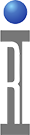DC Measurements on Cassini

The following table shows the different DC supplies on the Cassini and their capability. These supplies are located in the “Device Power” (RI8546B) TIM.

The “static digital” (DB) consists of 16 control lines which can be multiplexed between two low current supplies. There are also 8 medium current supplies known as the “dut control”(DP) where DP1-8 can be muxed to Vcc3&4, DP9-16 muxed to Vcc1&2, and Vcc5,6,7,and 8 are independent supplies with sense capability. The dut control also consists of four independent voltage measure (VM) pins for measuring voltage only. There are also two high current supplies with sense lines known as VI1 and VI2.

The current measure maximum and limit are defined by the ranges set in the table below. For example, the DPVP uses one of the four range values: ±2uA,±20uA,±200uA,±2mA. Any value can be set, but it will default to the next highest range value.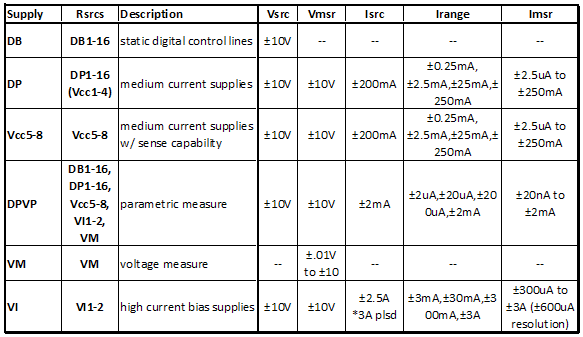*In order for to use VI1-2 with the paramteric measure, the hardware needs to consist of an updated module and the latest patch 86: August 09 updates
*In order for to use Vcc5-8 with the paramteric measure, you need the latest patch 86: August 09 updates

The following is outlined in further detail below:

I. Current Measurements Using a DP/Vcc/VI
II. Force Voltage and Measure Current Using a DP
III. Force Voltage and Measure Current Using a DPVP
IV. Voltage Measurements Using a DP/Vcc/VI
V. Force Current and Measure Voltage Using a DP
VI. Force Current and Measure Voltage Using a DPVP
VII. Draw Current and Measure Voltage Using a DPVP
I. Current Measurements Using a DP/Vcc/VI:
Below are examples on how to do current measurements using the medium and high current supplies.

1.1 ) Imeasure with DP: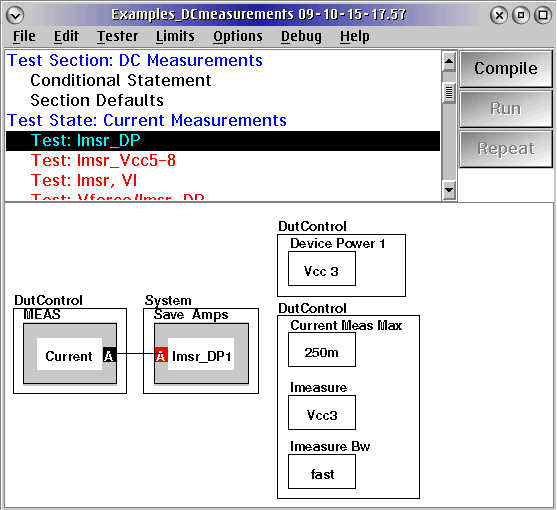1.2) Imeasure with Vcc5-8: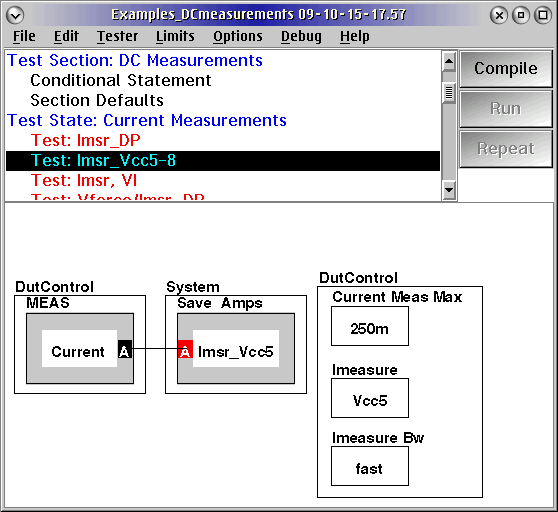1.3) Imeasure with Power VI: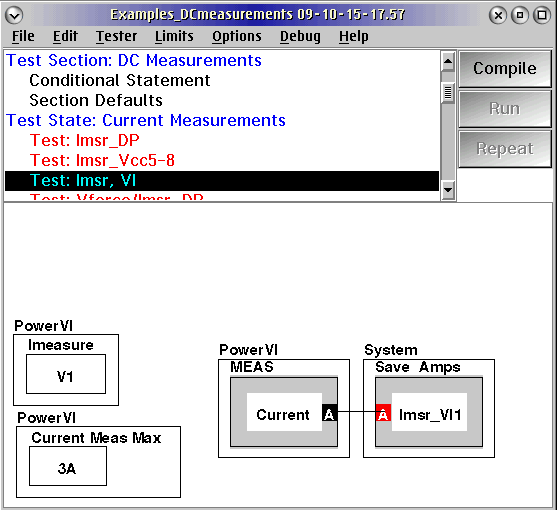II. Force Voltage and Measure Current Using a DP:
Outlined below are the methods for forcing voltage and measuring current using a DP resource, such as DP1-16 with Vcc1-4.

2.1) Vforce, Imeasure with DP:

This shows the standard approach for setting a voltage on a DP line and measuring the current. The setup buttons are on the left which sets DP1 (using Vcc4) to 1V and the measurement buttons are on the right side of the panel, which defines the measurement pin as Vcc4 and sets a limit of 250mA max.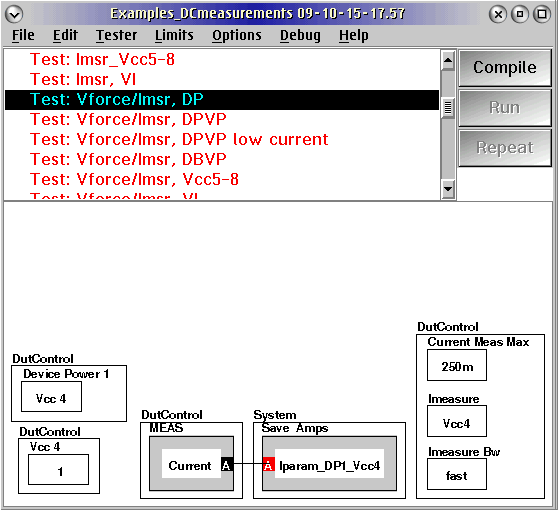III. Force Voltage and Measure Current Using a DPVP:

The parametric measure (DPVP) is used to force voltage and measure current or force current and measure voltage for currents ≤2mA. A DB, DP, VI, or VM can be switched to the DPVP in order to do parametric measurements. There is also a low current option which provides nano-amp resolution.

All of these methods below are set up for a “make before break” situation to keep the part from losing voltage when switching to the DPVP. It is always good practice to initially set the “measure pin” which connects the DPVP to the desired resource, and then change that resource to “precision” or “open” in order to open the relay from one of the middle or high current supplies that it is originally connected to. The order is defined in a pre-measure and on completion of test, the order is reversed in the post-measure.

3.2) Vforce, Imeasure with DPVP_DP:

The image below shows the setup for forcing voltage and measuring current using the parametric measure (DPVP) with DP4 (which was originally connected to Vcc3). The setup is on the right-hand side of the panel and is done first. It shows that DP4 is set to Vcc3 which is set to 1V and the parametric measure is also set to 1V with a limit and maximum on current of 2mA. Next, the pre-measure is done and the “Measure Pin” connects the DPVP to DP4. After 100us, DP4 is set to “precision” which opens the relay from DP4 to Vcc3, so DP4 is then solely connected to the parametric measure. Then the measurement can be made and in the post-measure the connection is reset back to using Vcc3.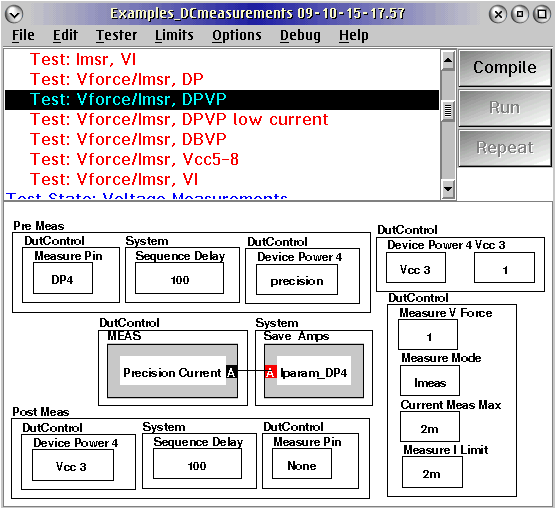3.3) Vforce, Imeasure LOW CURRENT with DPVP_DP:

This measurement is similar to the procedure outlined above in 3.2) Vforce, Imeasure with DPVP_DP; however, there is an added “low current” button which supports nano-amp resolution. However, this measurement will be slower.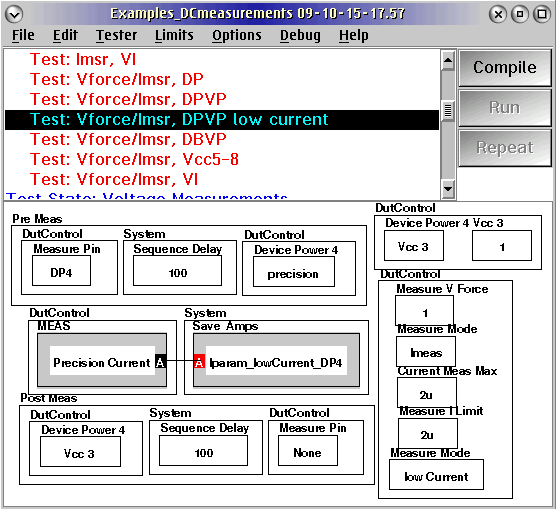3.4) Vforce, Imeasure with DPVP_DB:

Below is the setup for forcing voltage and measuring current using the parametric measure (DPVP) with static digital pin DB1.The setup is on the right-hand side of the panel and is done first. It shows DB1 is set to 1V and the parametric measure is also set to 1V with a limit and maximum on current of 2mA. Next, the pre-measure is done and the “Measure Pin” connects the DPVP to DB1 (Note, the “measure pin” is a static digital button). After 100us, the relay opens from DB1 to VON, so DB1 is then solely connected to the parametric measure. Then the measurement can be made and in the post-measure the connection is reset back to using VON.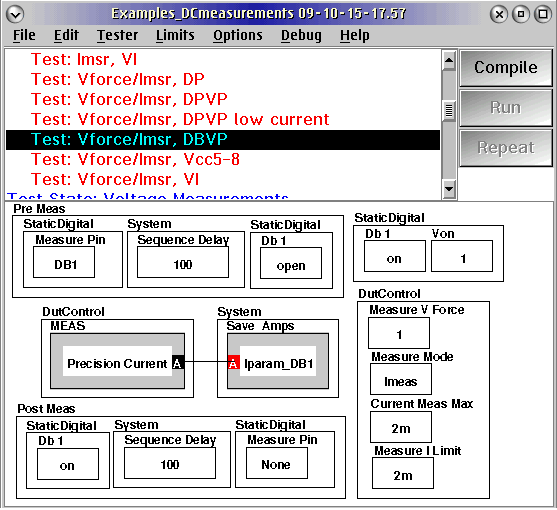3.5) Vforce, Imeasure with DPVP_Vcc5-8:

The image below shows the setup for forcing voltage and measuring current using the parametric measure (DPVP) with Vcc5-8 (Note, the “Vcc measure pin” is a separate dut control button). The setup is on the right-hand side of the panel and is done first. It shows that DP5 is set to ON which is set to 1V and the parametric measure is also set to 1V with a limit and maximum on current of 2mA. Next, the pre-measure is done and the “Measure Pin” connects the DPVP to Vcc5. After 100us, Vcc5 is set to “off” which opens the relay from Vcc5, so Vcc5 is then solely connected to the parametric measure. Then the measurement can be made and in the post-measure the connection is reset back to using Vcc5.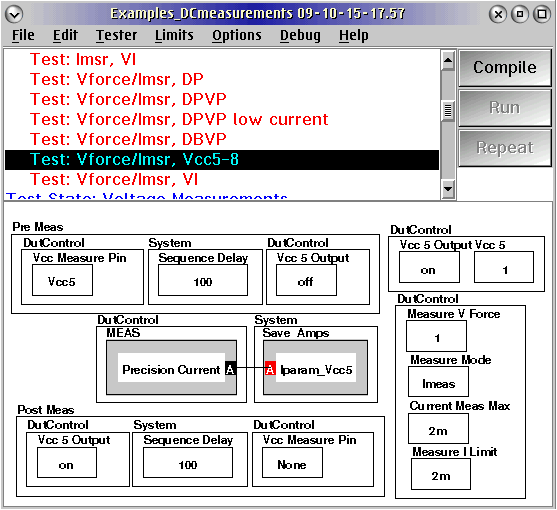3.6) Vforce, Imeasure with DPVP_Power VI:

The setup below illustrates how to force voltage and measure current using the parametric measure (DPVP) with VI1. The setup is on the right-hand side of the panel and is done first. It shows that VI1 is set to ON at 1V and the parametric measure is also set to 1V with a limit and maximum on current of 2mA. Next, the pre-measure is done and the "Measure Pin" connects the DPVP to VI1. After 100us, VI1 is set to "off" which opens the relay from VI1, so that it is then solely connected to the parametric measure. Then the measurement can be made and in the post-measure the connection is reset back to using VI1.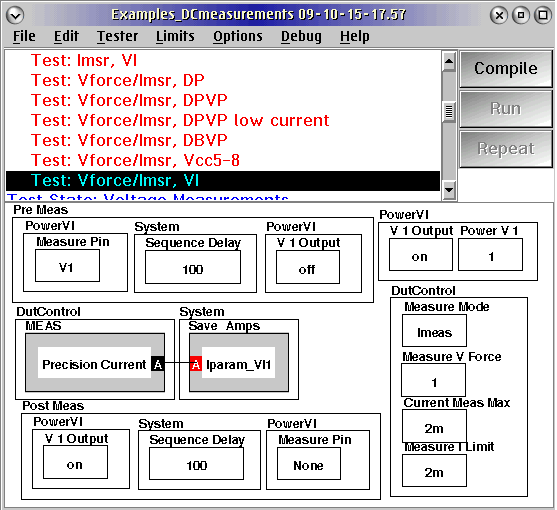*Note: When setting the current limit on the VI, try to set it to 99mA or less (where permitable) to keep from enduring a 40msec delay that is put in to protect from overloading the relay when switching from high current to a low current measurement.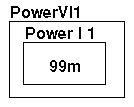IV.Voltage Measurements Using a DP/Vcc/VI:
Below are examples on how to do voltage measurements using the medium and high current supplies (DP,Vcc,VI) and the exclusive Vmeasure pins. These tests only require a resource pin and a maximum voltage defined.

4.1) Vmeasure with DP: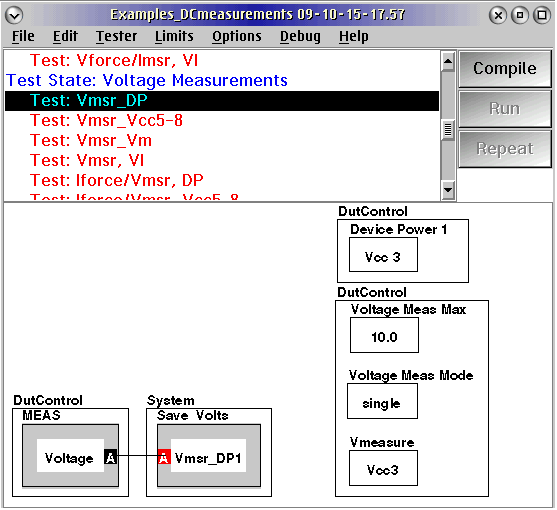4.2) Vmeasure with Vcc5-8: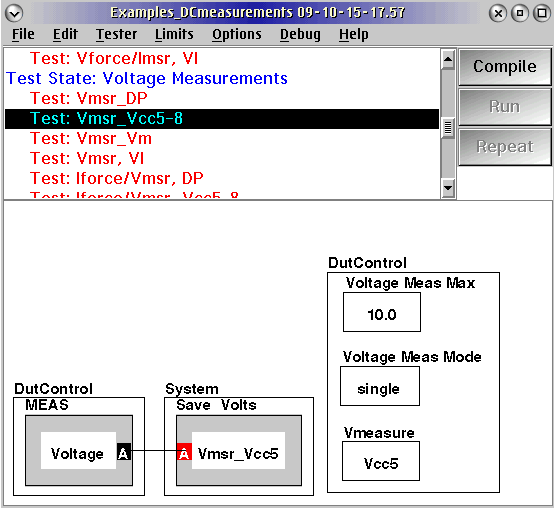4.3) Vmeasure with VM: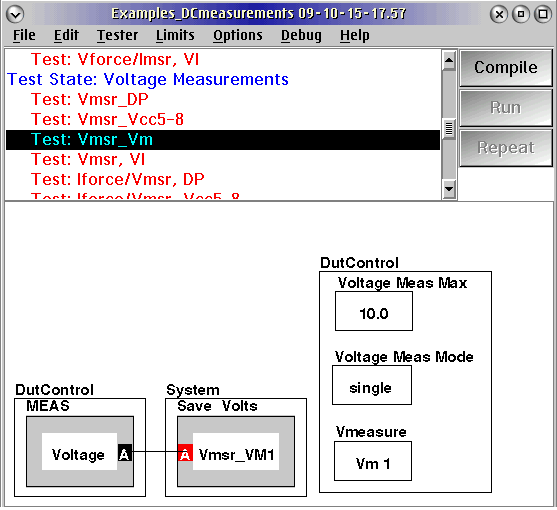4.4) Vmeasure with Power VI: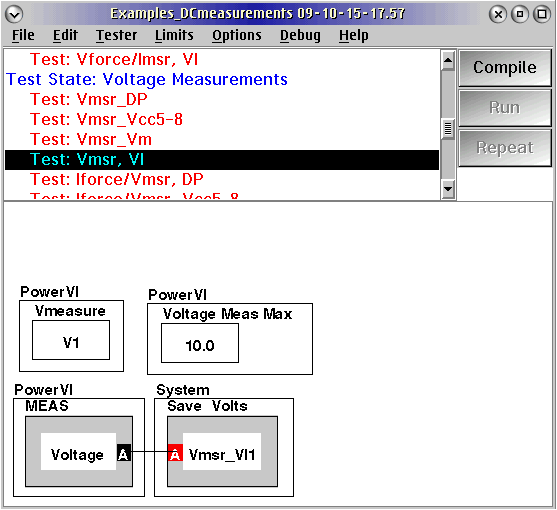V. Force Current and Measure Voltage Using a DP:
The following are examples of how to force current and measure voltage using the medium current supplies: Vcc1-8. The first example is for a dut control (DP) resource which use one of the supplies from Vcc1-4, and the second example uses a Vcc6-8 supply which is another medium current supply with sense capability.

The main point of concern is that you will want to prevent sharp current spikes by setting the sequence properly. In order to do this, you should initially set up the supply for current mode but with the current set to 0A. There should also be a minimum and maximum voltage set on the supply as well. Then once the setup is complete, increase the sourced current in a pre-measure and set it back to zero in a post-measure.

5.1) Iforce, Vmeasure with DP:

The example below shows the setup buttons on the left and the measure buttons on the right. Initially DP4 is set to Vcc3 in current mode. There is a maximum voltage of 1.8V and a minimum of 0V on the Vcc3 supply. The measurement pin is defined as Vcc3 on the right with a measurement cap of 4V. After this setup is completedVcc3 will be sourced 100mA and the measurement will be made. After completion of the measurement, the current on Vcc3 will be turned off or set to 0A.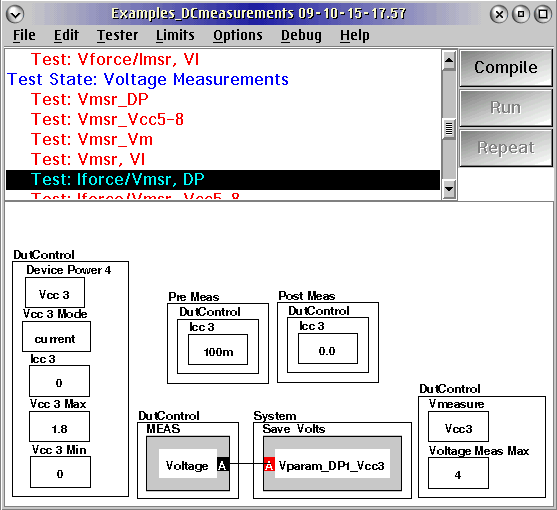5.2) Iforce, Vmeasure with Vcc5-8:

This example is similar to that defined in the procedure prior to this one. Similarly, the setup supply buttons are on the left and the setup measure buttons on the right. Initially Vcc5 is set to current mode with 0A and a maximum voltage of 1V and a minimum of 0V. On the right hand side the measurement pin is defined as Vcc5 with a measurement limit of 10V. After this setup is completedVcc5 will source 100mA and the measurement will be made. After completion of the measurement, the current on Vcc3 will be turned off or set to 0A.VI. Force Current and Measure Voltage Using a DPVP:

The parametric measure (DPVP) is used to force voltage and measure current or force current and measure voltage for currents ≤2mA. A DB, DP, VI, or VM can be switched to the DPVP in order to do parametric measurements.

When using the DPVP be sure to set the device power, static digital, and Vcc measure pin buttons to “none” in the global defaults. This will keep the DPVP disconnected when not in use which will further prevent any connection conflicts to the DPVP.

6.1) Iforce, Vmeasure with DPVP_DB:

Below is the setup for forcing current and measuring voltage using the parametric measure (DPVP) with static digital pin DB1.The panel shows forcing 10uA on DB1, while setting the measurement to voltage with a 10A limit (Note, the “measure pin” is a static digital button).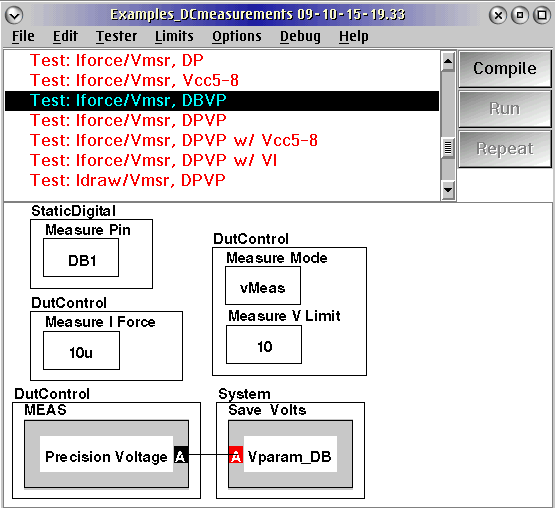6.2) Iforce, Vmeasure with DPVP_DP:

The image below shows the setup for forcing current and measuring voltage using the parametric measure (DPVP) with DP1. DP1 is switched to the parametric measure by setting it to “precision”, and 10uA is being supplied to this resource. The mode is set to “vMeas” for voltage with a 10V limit on the measure.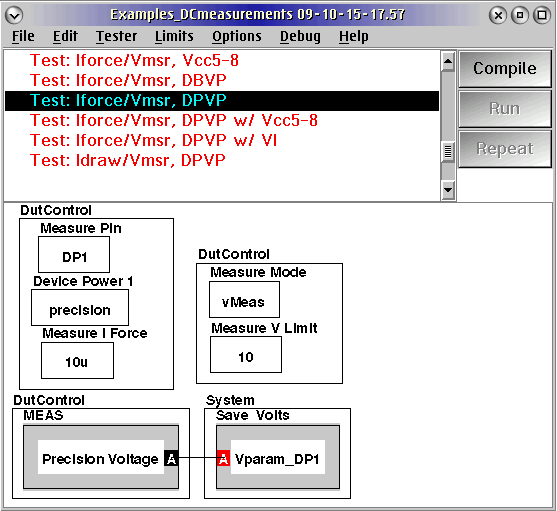6.3) Iforce, Vmeasure with DPVP_Vcc5-8:

The image below shows the setup for forcing 10uA on Vcc5 and measuring voltage with a 10V limit (Note, the “Vcc Measure Pin” is a separate dut control button).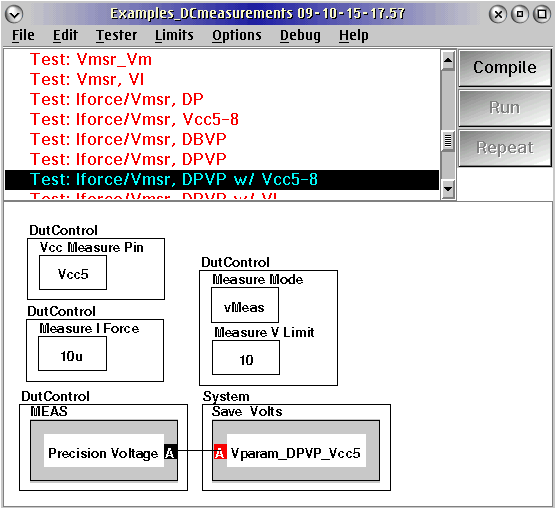6.4) Iforce, Vmeasure with DPVP_VI:

The image below shows the setup for forcing 10uA on VI1 and measuring voltage with a 10V limit (Note, the “Measure Pin” is a separate Power VI button).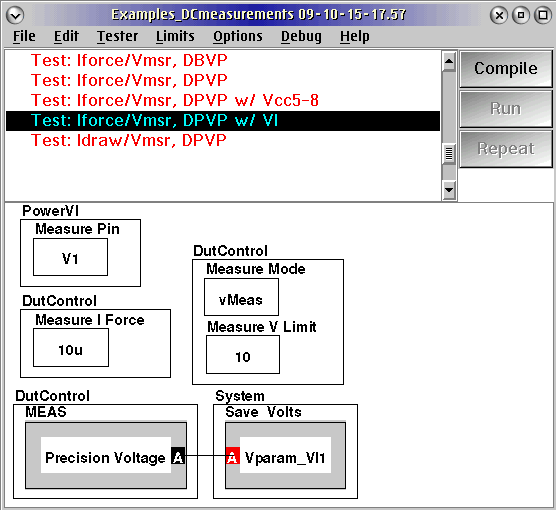VII. Draw Current and Measure Voltage Using a DPVP:

7.1) Idraw, Vmeasure with DPVP:

This test is similar to the procedure described above for forcing current and measuring voltage using the DPVP; however, the Iforce = -10uA to draw current instead of +10uA to force current. Also, the limit on the voltage (Vlimit) needs to be set to a value less than what you are expecting. The measurement expects that you will be measuring a negative voltage (such as -2V), so the limit value expected would be something less than that (such as -4V). So, the case is the same for if you are expecting to measure a positive voltage with a current draw, such as +2V, then you would still have to set a limit to 0V or something less than +2V to say that you don’t want a value to be less than X volts.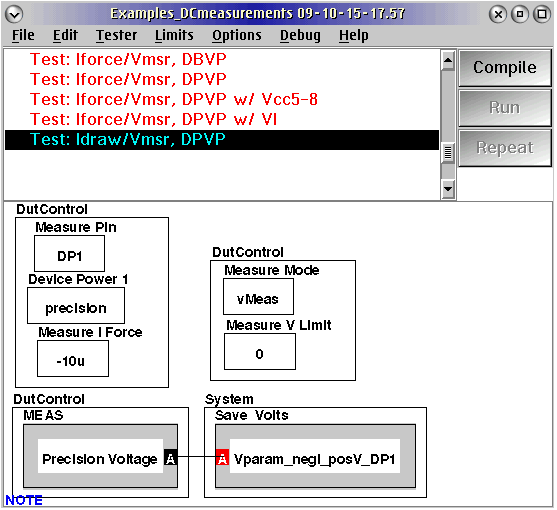https://roos.com/docs/JLUT-7UQ4KK
ROOS INSTRUMENTS CONFIDENTIAL AND PROPRIETARY